|
|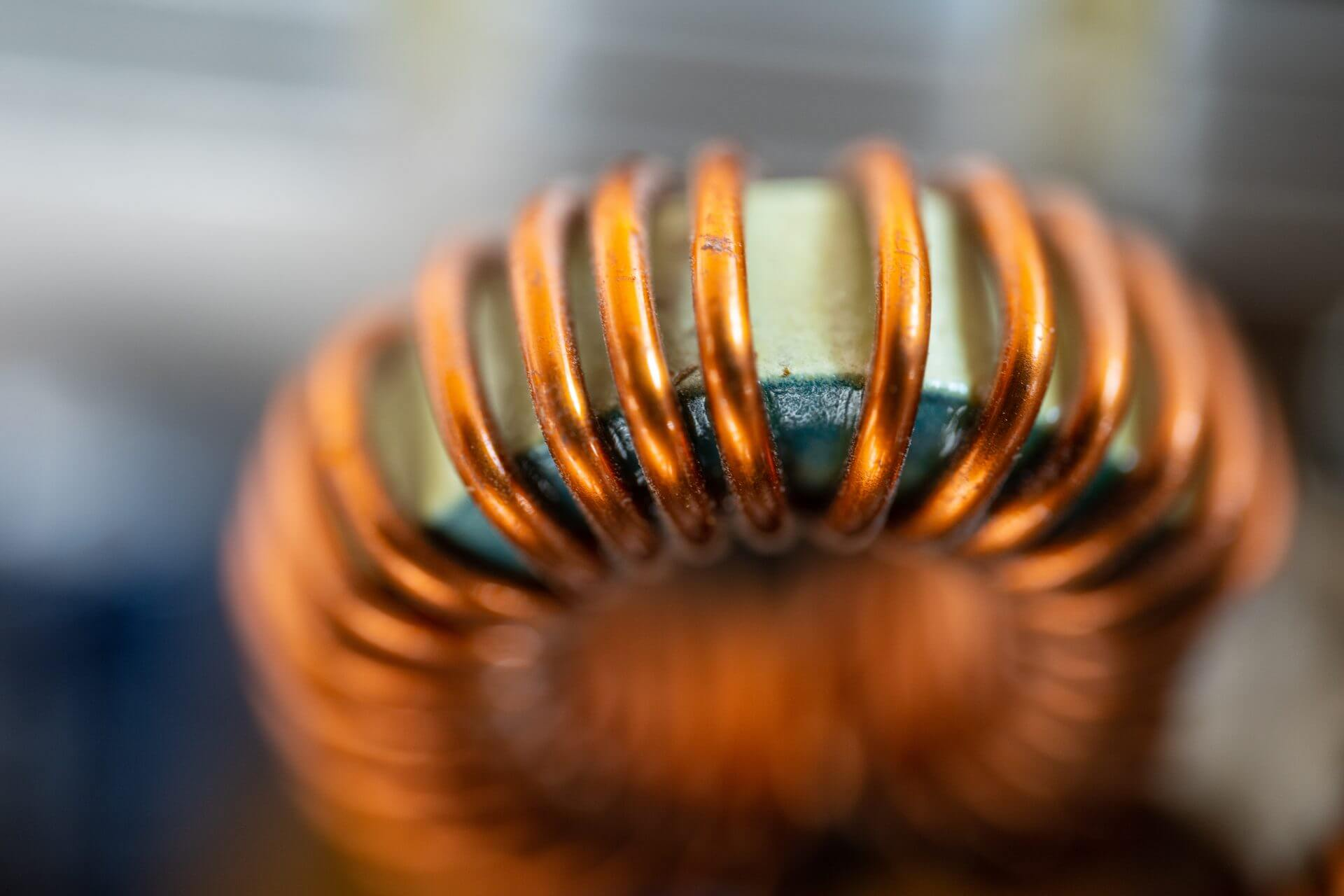### How Can I Use an Inductor and a Capacitor for Impedance Matching?

March 22, 2023

For the simplest case of real input, Rin, to real output impedance Rout, there are two possibilities: Rin > Rout or Rin < Rout. The two L topologies to accomplish this are: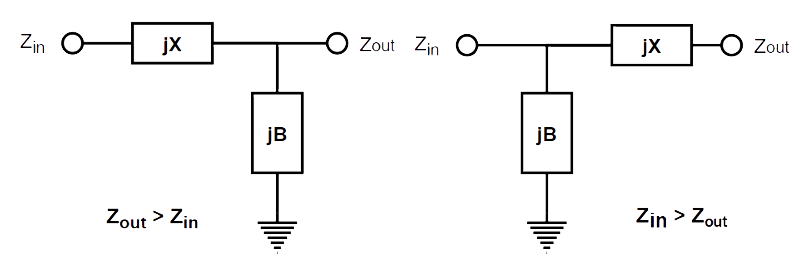Figure 1 – Matching Topologies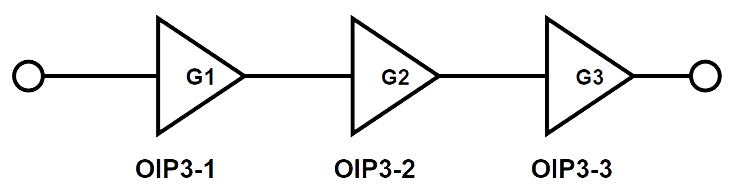Figure 2 – Amplifier Chain

If we assume the Zout is real R2, Zin is real R1, and R2 > R1 (the left-side topology in Figure 2) we can use the Norton transformation to get: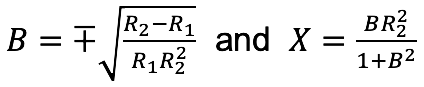For example, to match R1 = 50Ω to R2 =1000Ω at 100 MHz: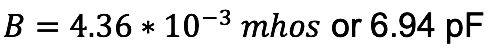choosing the positive root to use a shunt capacitor for a lowpass implementation, then: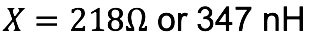For R2 < R1, simply swap R2 for R1 in the above calculations. The useful bandwidth of the match will depend on the ratio of R2 to R1. Extreme transformations result in very narrow bandwidth results. A broader band match may be implemented using more L-C elements  .

See this and more important radio frequency charts and formulas in this comprehensive application note

 G.L. Matthaei, “Tables of Chebyshev impedance–transforming networks of low-pass filter form”, Proceedings of the IEEE, Vol 52, Issue 8

 R.M. Fano, “Theoretical limitations on the broadband matching of arbitrary impedances”, Journal of the Franklin Institute, Vol 249, Jan 1950, pp 57-83